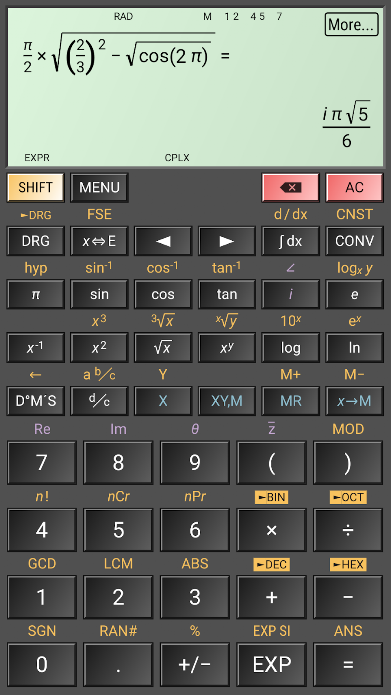#### HiPER Scientific Calculator

HiPER Scientific Calculator is a popular calculator with more than 16 million downloads and 100,000 five-star ratings.

(Android OS)The calculator has many functions, such as:

– basic arithmetic operations including percentage, modulo and negation;
– fractions (in the expression mode any expression including nested fractions can be entered as a numerator and a denominator);
– mixed numbers;
– periodic numbers and their conversion to fractions;
– unlimited number of braces;
– operator priority;
– repeated operations;
– variables and symbolic computation;
– derivatives and integrals;
– graphs of functions and integral area;
– calculation details – extended information about a calculation like all complex roots, unit circle etc.;
– complex numbers
– conversion between rectangular and polar coordinates
– advanced number operations such as random numbers, combinations, permutations, common greatest divisor, etc.;
– trigonometric and hyperbolic functions;
– powers, roots, logarithms, etc.;
– degrees, minutes and seconds conversion;
– fixed point, scientific and engineering display format;
– display exponent as SI units prefix;
– memory operations with 10 extended memories;
– clipboard operations with various clipboard formats;
– result history;
– binary, octal and hexadecimal numeral systems;
– logical operations;
– bitwise shifts and rotations;
– haptic feedback;
– more than 90 physical constants;
– conversion among 250 units;
– Reverse Polish notation.